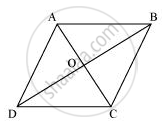# Show that If the Diagonals of a Quadrilateral Bisect Each Other at Right Angles, Then It is a Rhombus. - Mathematics

Show that if the diagonals of a quadrilateral bisect each other at right angles, then it is a rhombus.

#### SolutionLet ABCD be a quadrilateral, whose diagonals AC and BD bisect each other at right angle i.e., OA = OC, OB = OD, and ∠AOB = ∠BOC = ∠COD = ∠AOD = 90º. To prove ABCD a rhombus, we have to prove ABCD is a parallelogram and all the sides of ABCD are equal.

In ΔAOD and ΔCOD,

OA = OC (Diagonals bisect each other)

∠AOD = ∠COD (Given)

OD = OD (Common)

∴ ΔAOD ≅ ΔCOD (By SAS congruence rule)

Similarly, it can be proved that

AD = AB and CD = BC (2)

From equations (1) and (2),

AB = BC = CD = AD

Since opposite sides of quadrilateral ABCD are equal, it can be said that ABCD is a parallelogram. Since all sides of a parallelogram ABCD are equal, it can be said that ABCD is a rhombus.

Concept: Another Condition for a Quadrilateral to Be a Parallelogram
Is there an error in this question or solution?
Chapter 8: Quadrilaterals - Exercise 8.1 [Page 146]

#### APPEARS IN

NCERT Class 9 Maths